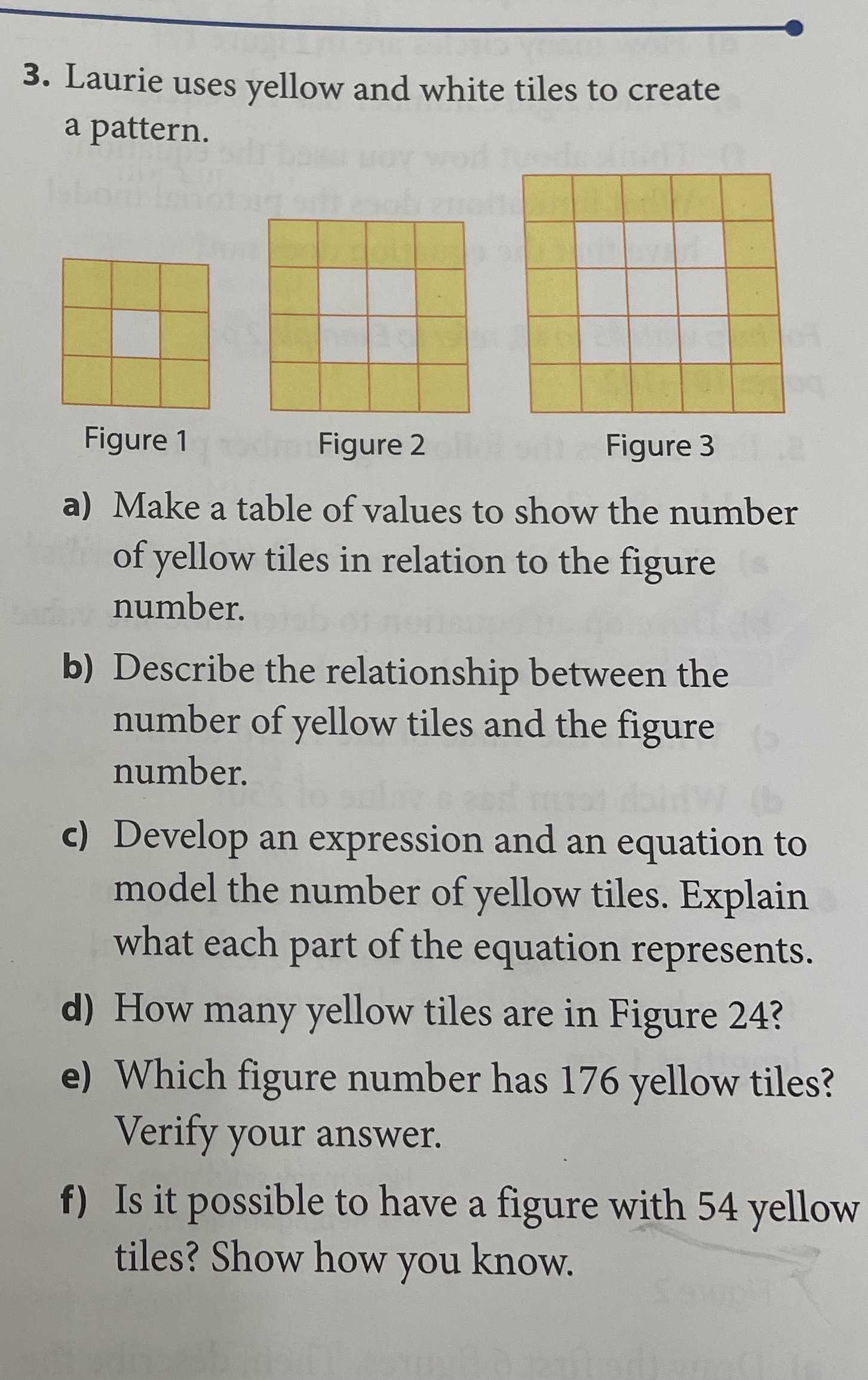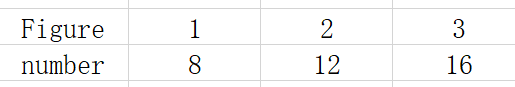### ¿Todavía tienes preguntas de matemáticas?

Pregunte a nuestros tutores expertos
Algebra
Pregunta3. Laurie uses yellow and white tiles to create a pattern.

a) Make a table of values to show the number of yellow tiles in relation to the figure number.

b) Describe the relationship between the number of yellow tiles and the figure number.

c) Develop an expression and an equation to model the number of yellow tiles. Explain what each part of the equation represents.

d) How many yellow tiles are in Figure $$24$$ ?

e) Which figure number has $$176$$ yellow tiles? Verify your answer.

f) Is it possible to have a figure with $$54$$ yellow tiles? Show how you know.

a)b)The difference between each picture and the previous one is 4

c)$$a_{n}= 4n+ 4$$

d)100

e)43

f)no

Solución
View full explanation on CameraMath App.